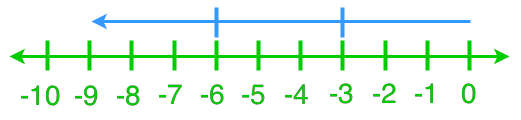# Dividing integers#### All You Need in One Place

Everything you need for better marks in primary, GCSE, and A-level classes.#### Learn with Confidence

We’ve mastered the UK’s national curriculum so you can study with confidence.#### Instant and Unlimited Help

0/1
##### Intros
###### Lessons
1. Introduction to division statements with non-integer quotients
0/16
##### Examples
###### Lessons
1. Understanding Division Using a Number Line
What are the two division statements that each diagram represents?

1.2.2. Solving Division Statements Using a Number Line
Use a number line to find each quotient.
1. (+10)÷(+5)
2. (+8)÷(-2)
3. (-12)÷(+4)
4. (-9)÷(-3)
3. Division Statements With Integer Quotients
Solve.
1. (+36)÷(-6)
2. (-45)÷(+15)
3. (-28)÷(-4)
4. 0÷(-16)
4. Division Statements With Non-Integer Quotients
Solve.
1. (-36)÷(+8)
2. (-100)÷(-3)
3. (+47)÷(+6)
4. (+34)÷(-7)
5. Word Problems of Dividing Integers
Katie participated in a track competition. If her average sprinting speed is 8 m/s, how long does it take for her to finish an 100 m sprint?
1. Peter finished a 45 km long cycling trail. He first cycled for 3 hours and then, he took a break. Afterwards Peter cycled for another 2 hours to finish the trail. What was his cycling rate per hour? Were there any assumptions?
0%
##### Practice
###### Topic Notes
In this section, we will keep working on dividing integers. We will learn how to read and represent division statements on a number line. We will then practice more integer divisions with actual numbers and word problems.

In this lesson, we will learn:

• Understanding Division Using a Number Line
• Solving Division Statements Using a Number Line
• Division Statements With Integer Quotients
• Division Statements With Non-Integer Quotients
• Word Problems of Dividing Integers

Notes:
• Recurring/ repeating decimals: decimal numbers whose digits repeat forever.
• For the recurring decimals, we put either a bar or dots above the recurring parts.